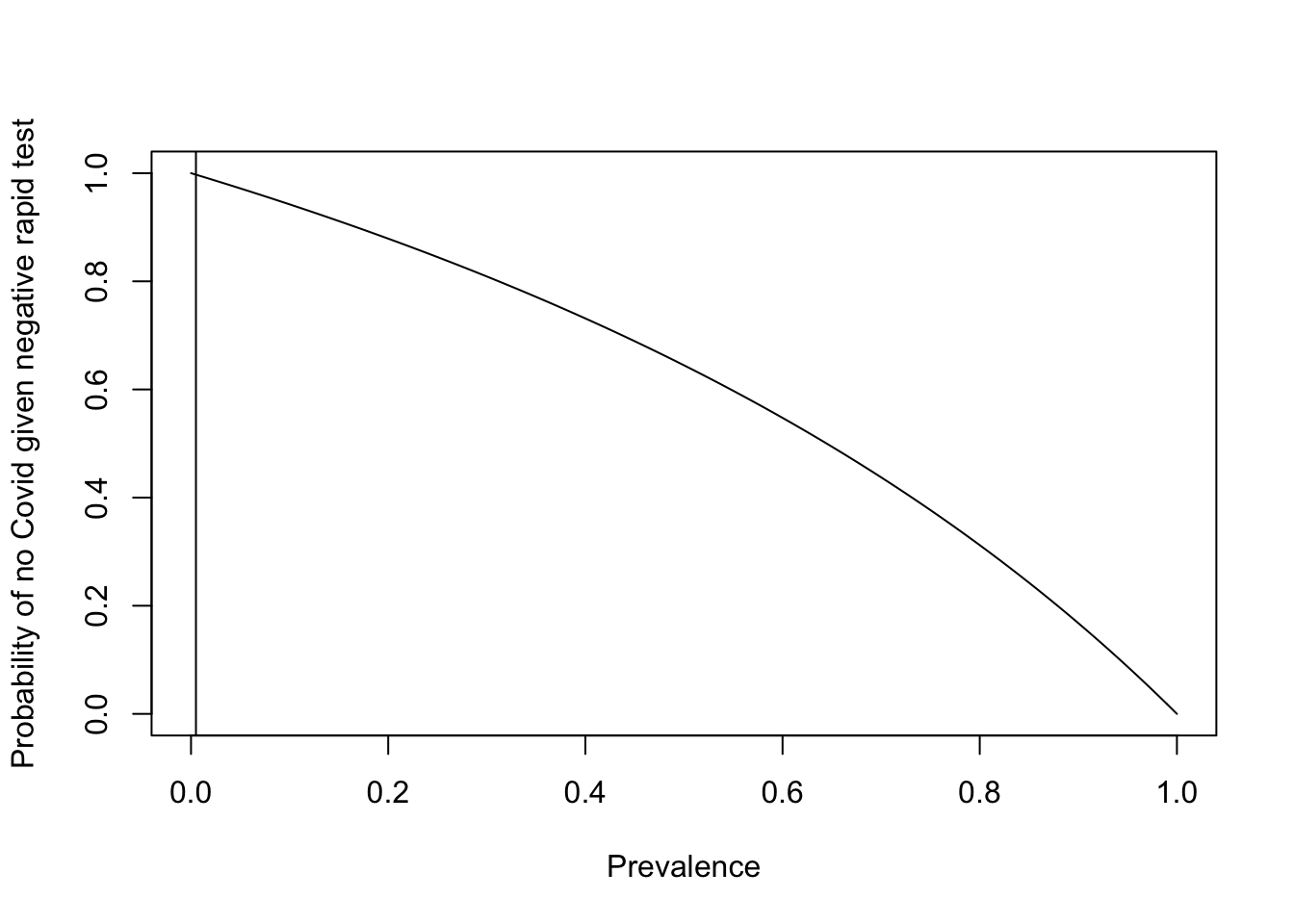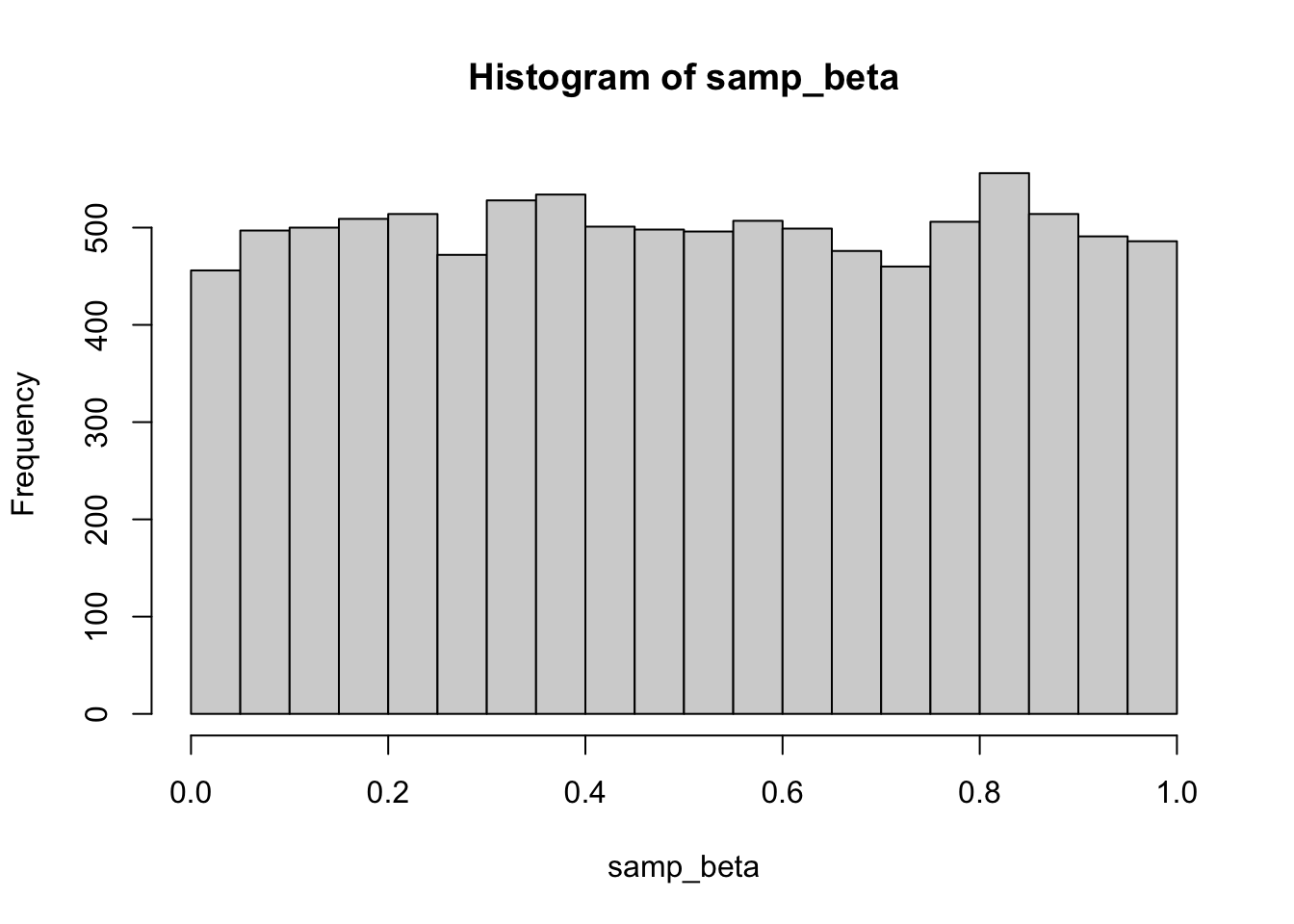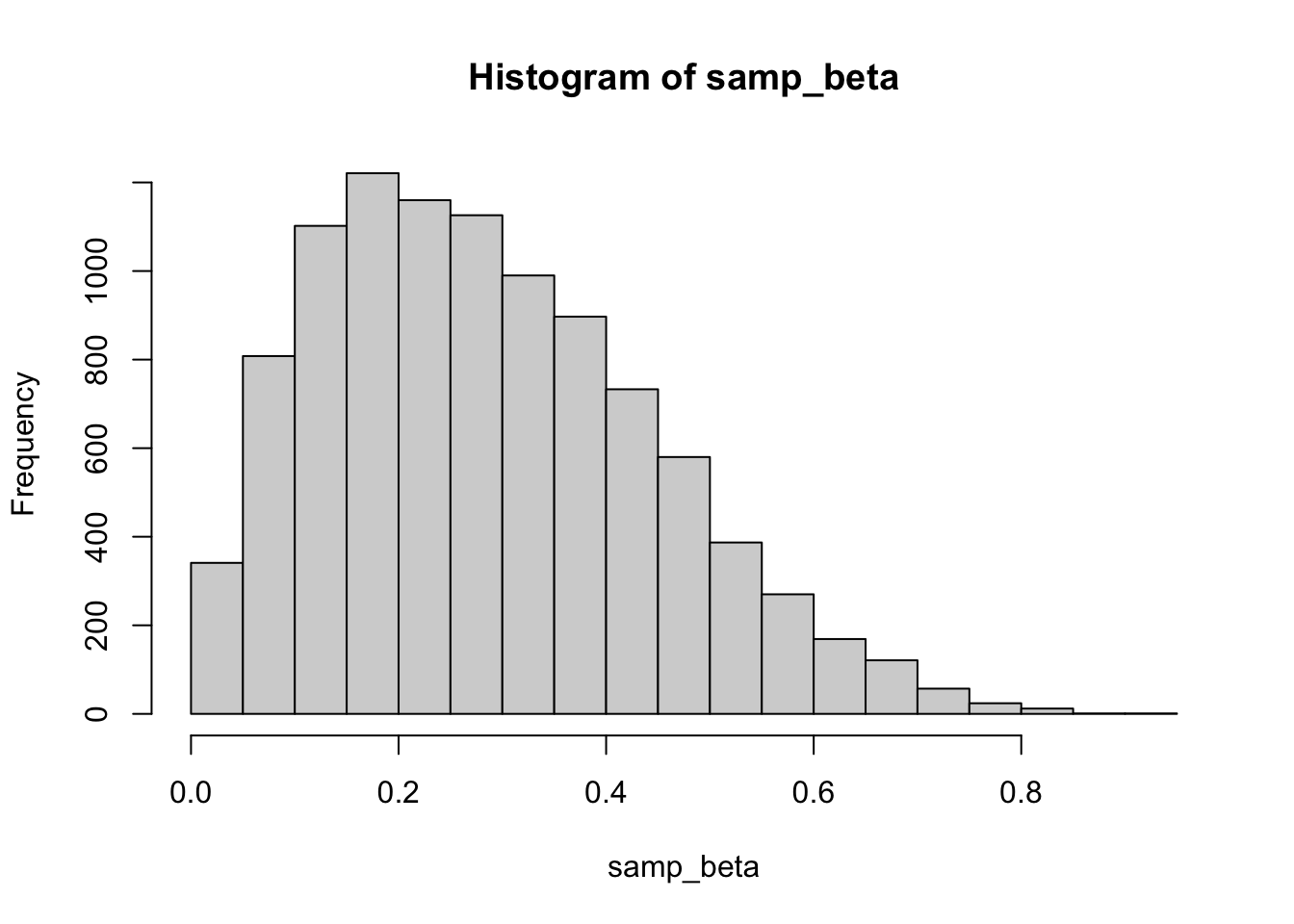## Overview

This document provides a brief introduction into Bayesian analysis and thinking. Some prerequisite knowledge in standard Frequentist statistics and modeling is helpful to understand the material. Nested within this tutorial are R code blocks that implement the new concepts.

### Outline

• Bayes’ Rule and Bayesian thinking
• Implementing Bayesian regression models in practice
• Workshop: A demonstration of these ideas via a re-analysis of the ART trial
• References and additional resources for learning Bayesian statistics

### Preliminary Setup

For the workshop component, we will use R locally. To be ready for this, you should have the brms package installed (and also the insight package). Feel free to try to install during the break. brms is a somewhat troublesome package at install - if you have issues you can troubleshoot here or just feel free to just watch my shared screen at that time.

## Bayesian Thinking vs. Frequentist Thinking

Before describing Bayesian thinking and philosophy, it is helpful to review the core tenets of Frequentist statistics. Frequentist probability is defined as the limit of an event’s relative frequency in a large number of repetitions. For example, if the probability of a power outage during this presentation is 0.01%, then if we were to repeat this presentation an infinite number of times, we would expect a power outage about 1 in every 10,000 presentations on average.

Statistical inference begins with the description of some unknown parameter of interest, which we’ll denote here as $$\theta$$. In Frequentist inference, often one will perform an analysis involving

1. Point estimation of $$\theta$$
2. Confidence interval calculation
3. Hypothesis testing

Point estimation entails calculating a “best guess” for $$\theta$$ according to some chosen definition of best guess. Then a confidence interval gives a range of estimates for $$\theta$$ where the confidence level (say 95%) can be interpreted as the long-run probability of a calculated interval containing the true parameter value.

Finally, one might specify a set of null hypothesis test and then calculate whether there us enough evidence to reject such a hypothesis using a p-value. For example, one might specify a null hypothesis that the mean number of cars owned by Americans is 0.5. Then one specifies a test statistic which is a function of a relevant data sample, taking into account sample mean, size, and variability. Then the calculated p-value represents the probability that, if the null is true, one would sample a test statistic as extreme as that calculated for the data. Even with this somewhat hand-wavy wording, it takes a lot of build-up to describe a p-value!

Now let’s contrast these ideas with the Bayesian paradigm. The Bayesian definition of a probability is that it is a quantification of belief that something will occur. Bayesian inference is undergone by combining sample data with any known prior information to learn about $$\theta$$. Point estimation is also common in Bayesian inference. Instead of confidence intervals, one would typically calculate a $$C$$% credible interval, for which the probability that $$\theta$$ lies within the interval is $$C$$%. One does not typically perform hypothesis testing in the Bayesian framework, and instead uses the posterior output (calculating using the observed data and prior information) to answer questions of interest about $$\theta$$ directly. So in the example above, one might just directly calculate the probability that the mean number of cars owned by Americans is greater than 0.5 given observed data and any prior known information.

In general, Frequentist methods will try to learn about the probability of the observed data (denoted throughout at $$y$$) given some parameter value, while Bayesian methods will try to learn about the probability of a parameter value given the observed data.

Many of these ideas are somewhat vague until we do concrete examples. Let’s define some basic tools for Bayesian inference and then provide some examples.

### Bayes’ Rule

Bayes’ Rule provides a means to calculate a posterior distribution for the parameter of interest - a distribution that updates our prior beliefs using the observed data.

Before discussing Bayes’ rule, it is helpful to review some conditional probability rules. Let $$A$$ and $$B$$ be events. Let $$P(A)$$ be the probability that event $$A$$ occurs and let $$P(A|B)$$ be the probability that event $$A$$ occurs, given that event $$B$$ has occurred. Then $\begin{equation*} P(A | B) = \frac{P(A, B)}{P(B)} \hspace{2cm} P(B | A) = \frac{P(A, B)}{P(A)} \end{equation*}$

If the joint probability $$P(A, B)$$ is not known, you can use one of these quantities to calculate the other. For example, $$P(B|A)P(A) = P(A, B)$$, and thus, $\begin{equation*} P(A | B) = \frac{P(B | A)P(A)}{P(B)} \end{equation*}$

The above equation is also known as Bayes’ Rule or Bayes Theorem. Going back to the setting of learning about population parameters using data, we can state the rule as $\begin{equation*} p(\theta | y) = \frac{p(y | \theta)p(\theta)}{p(y)} \end{equation*}$ where $$p()$$ is shorthand for either probability or density functions for simplicity.

The term $$p(\theta)$$ reflects prior knowledge about the parameter of interest. The term $$p(y|\theta)$$ can be modeled using standard approaches, and the term $$p(y)$$ is often not needed, for reasons that will be described later. Let’s ground this idea with some examples.

### Example 1

John just took a Covid rapid test and it came back negative. The test instructions say that the probability of a negative test given that you truly don’t have Covid is 99.8%. They also say that the probability of a positive test given that you do have Covid is 45%. But John wants to know the probability that he doesn’t have Covid given this new result, which they didn’t provide. Let’s calculate this quantity using a simple application of Bayes’ Theorem.

\begin{align*} P(\text{Not Covid | Negative}) &= \frac{P(\text{Negative | Not Covid})P(\text{Not Covid})}{P(\text{Negative})} \\ &= \frac{P(\text{Negative | Not Covid})P(\text{Not Covid})}{P(\text{Negative, Not Covid}) + P(\text{Negative, Covid})} \\ &= \frac{P(\text{Negative | Not Covid})P(\text{Not Covid})}{P(\text{Negative | Not Covid})P(\text{Not Covid}) + P(\text{Negative | Covid})P(\text{Covid})} \\ &= \frac{0.998P(\text{Not Covid})}{0.998P(\text{Not Covid}) + 0.55P(\text{Covid})} \end{align*}

Thus the solution is a function of a prior probability that you had Covid. In disease contexts, this prior is often replaced by the prelavence in a particular area, for example the current prevalence of Covid in Philadelphia. Let’s plot this function in R:

prev_list <- seq(0, 1, 0.01)
val_list <- (0.998*(1-prev_list)) / ( (0.998*(1-prev_list)) + 0.55*prev_list )
plot(prev_list, val_list, type = "l", xlab = "Prevalence", ylab = "Probability of no Covid given negative rapid test")
abline(v = 0.005)John heard that the current prevalence in Philly is about 0.5%, so he’s pretty confident that he does not have Covid.

### Example 2

Suppose a new Covid wave is starting and Mary is interested in the prevalence of infection in the city of Philadelphia, denoted $$\theta$$, and she plans to work remotely if the prevalence is greater than 4%. She collects a sample of PCR tests from 50 random individuals from the city and 4 of them are positive.

There are a couple of ways to do a Frequentist analysis for the parameter of interest. An unbiased estimate of the prevalence is given by the sample mean $$\hat{\theta} = 0.08$$ and a standard approach for calculating a confidence interval (without small sample correction) yields an interval of (0.005, 0.155). If Mary wants to use one-sided hypothesis testing with $$\alpha = 0.05$$ to make a decision of whether the prevalence is greater than 4%, then she gets $$p=0.14$$ and fails to reject the null hypothesis (here using an exact test because of the small sample).

binom.test(4, 50, p = 0.04, alternative = "greater")
##
##  Exact binomial test
##
## data:  4 and 50
## number of successes = 4, number of trials = 50, p-value = 0.1391
## alternative hypothesis: true probability of success is greater than 0.04
## 95 percent confidence interval:
##  0.02778767 1.00000000
## sample estimates:
## probability of success
##                   0.08

Bayesian thinking provides two critical benefits in this type of analysis:

1. While hypothesis testing is useful in many scenarios, it can often be overly stringent (or arbitrary with respect to the choice of $$\alpha$$) and you may want to make decisions with a more holistic process.
2. Bayesian analysis gives us the opportunity to leverage prior information.

Let’s start with just the second point. Suppose that before Mary runs her analysis, a colleague tells her that the estimated prevalence in New York City, Baltimore, and Pittsburgh are 0.12, 0.06, and 0.03 respectively. The first step to Bayesian analysis is to encode this information into a prior distribution, $$p(\theta)$$.

We want to use a distribution family that takes values from 0 to 1, the support of our parameter of interest. A logical choice is the Beta distribution. Using the information on nearby cities, it seems probable but not guaranteed that the prevalence in Philly is between 0.03 and 0.12. So we should pick a Beta prior with parameters that put most, but not all, of the distribution within that range.

Let’s find such a distribution using R. One way to do this is by changing the shape1 and shape2 parameters until you find a distribution that has the desired properties.

samp_beta <- rbeta(10000, shape1 = 1, shape2 = 1)
hist(samp_beta)samp_beta <- rbeta(10000, shape1 = 2, shape2 = 5)
hist(samp_beta)samp_beta <- rbeta(10000, shape1 = 5, shape2 = 2)
hist(samp_beta)